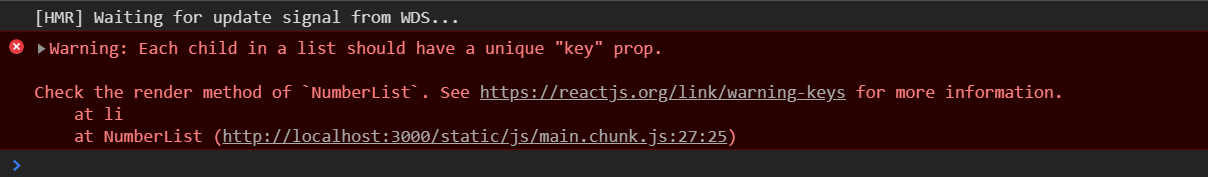### list 列表和 key

``````const numbers = [1, 2, 3, 4, 5];
const double = numbers.map((number) => { return number * 2});
console.log(double)

//output:
//[ 2, 4, 6, 8, 10 ]``````

``````const numbers = [1, 2, 3, 4, 5];
const listItems = numbers.map((number) => <li>{number}</li>)

ReactDOM.render(
<ul>{listItems}</ul>,
document.getElementById('root')
);``````

``````const NumberList = (props) => {
const numbers = props.numbers;
const listItems = numbers.map((number) => <li>{number}</li>)
return (
<ul>{listItems}</ul>
)
}

const numbers = [1, 2, 3, 4, 5];
ReactDOM.render(
<NumberList numbers={numbers}/>,
document.getElementById('root')
);``````Key 是一个特殊的 string 字符串属性需要给创建的 list element 添加的。它可以用来定位 list 中的每个元素。

``````const NumberList = (props) => {
const numbers = props.numbers;
const listItems = numbers.map((number) =>
<li key={number.toString()}>
{number}
</li>);
return (
<ul>{listItems}</ul>
);
}``````

#### Keys

Key 可以帮助 React 识别哪个 item 修改过，被删除，被添加。以上示例中，我们在 map 中创建 item 时给其 key 属性，这样每个 item 可以有确切的属性值。

``````const TodoItems = (props) => {
const todos = props.todos;
const listItems = todos.map((todo) =>
<li key={todo.id}>
{todo.text}
</li>
)
return (
<ul>{listItems}</ul>
);
}
const todos = [
{id: 1, text: '123'},
{id: 2, text: '456'}
];
ReactDOM.render(
<TodoItems todos={todos} />,
document.getElementById('root')
);``````

``````const TodoItems = (props) => {
const todos = props.todos;
const listItems = todos.map((todo, index) =>
<li key={index}>
{todo.text}
</li>
)
return (
<ul>{listItems}</ul>
);
}``````

#### 拆解 component 时 key 的处理

keys 是对应与一个数组的内容而言的，它并不能单独存在。例如我们要拆解上面的 NumberList，提取出 ListItem，则需要将 key 定义在 `<ListItem />` 元素中而不是 ListItem component 内部的 `<li>` 中：

``````const ListItem = (props) => {
return (
<li>{props.value}</li>
);
}

const NumberList = (props) => {
const numbers = props.numbers;
const listItems = numbers.map((number) =>
<ListItem key={number.toString()} value={number}/>);
return (
<ul>{listItems}</ul>
);
}

const numbers = [1, 2, 3, 4, 5];
ReactDOM.render(
<NumberList numbers={numbers} />,
document.getElementById('root')
);``````

``````function ListItem(props) {
const value = props.value;
return (
<li key={value.toString()}>
{value}
</li>
);
}``````

#### 每个 item 的 key 必须是特定的

``````const React = require('react')
const ReactDOM = require('react-dom')

const Blog = (props) => {
const sideBar = (
<ul>
{props.posts.map((post) =>
<li key={post.id}>{post.title}</li>
)}
</ul>
);
const content = props.posts.map((post) =>
<div key={post.id}>
<h3>{post.title}</h3>
<p>{post.content}</p>
</div>
);
return (
<div>
{sideBar}
<hr/>
{content}
</div>
);
}

const posts = [
{id: 1, title: 'Hello World', content: 'Welcome to learning React!'},
{id: 2, title: 'Installation', content: 'You can install React from npm.'}
];

ReactDOM.render(
<Blog posts={posts} />,
document.getElementById('root')
);``````

key 是为了给 React 识别用的。它本身并不作为一个普通 prop 传给 components，也就是在 component 内部并不能使用这个 key 数据，如果想要在 component 中使用这个数据则需要单独定义一个其他 prop 来传入 key 数据：

``````const Post = (props) => {
return (
<li>
{props.id}: {props.title}
</li>
)
}
const Blog = (props) => {
const sideBar = (
<ul>
{props.posts.map((post) =>
<Post key={post.id} id={post.id} title={post.title} />
)}
</ul>
);
...
...
...
}``````

``````const NumberList = (props) => {
const numbers = props.numbers;
const listItems = numbers.map((number) =>
<ListItem key={number.toString()} value={number}/>);
return (
<ul>{listItems}</ul>
);
}``````

JSX 支持嵌入任何的 JavaScript 表达式，只需要使用大括号包围即可，所以上面的代码可以修改为以下模式：

``````    return (
<ul>
{numbers.map((number) =>
<ListItem key={number.toString()} value={number} />);}
</ul>
);``````

### Forms 表格

HTML 的 form element 和其他 DOM elements 有点区别，因为 form element 包含有一些内部 state 数据，例如下面的 html 示例包含一个 from 表格：

``````<form>
<label>
Name:
<input type="text" name="name">
</label>
<input type="submit" value="Submit">
</form>``````

### controlled components

``````const React = require('react')
const ReactDOM = require('react-dom')

class NameForm extends React.Component {
constructor(props) {
super(props);
this.state = {value: ''};

this.handleChange = this.handleChange.bind(this);
this.handleSubmit = this.handleSubmit.bind(this);
};

handleChange(event) {
this.setState({value: event.target.value});
}

handleSubmit(event) {
alert(`a name has been submited: \${this.state.value}`);
event.preventDefault();
}

render() {
return (
<form onSubmit={this.handleSubmit}>
<label>
Name:
<input type="text" value={this.state.value} onChange={this.handleChange} />
</label>
<input type="submit" value="Submit" />
</form>
);
}
}

ReactDOM.render(
<NameForm />,
document.getElementById('root')
);``````

#### textarea 标签

``````<textarea>
Hello there, this is some text in a text area
</textarea>``````

``````const React = require('react')
const ReactDOM = require('react-dom')

class NameForm extends React.Component {
constructor(props) {
super(props);
this.state = {value: 'please write some words to discribe yourself'};

this.handleChange = this.handleChange.bind(this);
this.handleSubmit = this.handleSubmit.bind(this);
};

handleChange(event) {
this.setState({value: event.target.value});
}

handleSubmit(event) {
alert(`a discribe has been submited: \${this.state.value}`);
event.preventDefault();
}

render() {
return (
<form onSubmit={this.handleSubmit}>
<label>
TextArea:
<textarea type="text" value={this.state.value} onChange={this.handleChange} />
</label>
<input type="submit" value="Submit" />
</form>
);
}
}

ReactDOM.render(
<NameForm />,
document.getElementById('root')
);``````

#### select 标签

``````<select>
<option value="grapefruit">Grapefruit</option>
<option value="lime">Lime</option>
<option selected value="coconut">Coconut</option>
<option value="mango">Mango</option>
</select>``````

``````const React = require('react')
const ReactDOM = require('react-dom')

class NameForm extends React.Component {
constructor(props) {
super(props);
this.state = {value: 'sports'};

this.handleChange = this.handleChange.bind(this);
this.handleSubmit = this.handleSubmit.bind(this);
};

handleChange(event) {
this.setState({value: event.target.value});
}

handleSubmit(event) {
event.preventDefault();
}

render() {
return (
<form onSubmit={this.handleSubmit}>
<label>
<select value={this.state.value} onChange={this.handleChange}>
<option value="sleep">Sleep</option>
<option value="sports">Sports</option>
<option value="takePhoto">Take Photo</option>
<option value="work">Work</option>
</select>
</label>
<input type="submit" value="Submit" />
</form>
);
}
}

ReactDOM.render(
<NameForm />,
document.getElementById('root')
);``````

``<select multiple={true} value={['B', 'C']}>``

### 处理多个 input 输入源

``````const React = require('react')
const ReactDOM = require('react-dom')

class NameForm extends React.Component {
constructor(props) {
super(props);
this.state = {
isGoing: true,
numberOfGuests: 2
};

this.handleChange = this.handleChange.bind(this);
this.handleSubmit = this.handleSubmit.bind(this);
};

handleChange(event) {
const target = event.target;
const value = target.type === 'checkbox' ? target.checked : target.value;
const name = target.name;
this.setState({[name]: value});
}

handleSubmit(event) {
alert(`Is Going: \${this.state.isGoing}, Number Of Guests: \${this.state.numberOfGuests}`);
event.preventDefault();
}

render() {
return (
<form onSubmit={this.handleSubmit}>
<label>
Is Going:
<input
name="isGoing"
type="checkbox"
checked={this.state.isGoing}
onChange={this.handleChange} />
</label>
<br />
<label>
Number of Guests:
<input
name="numberOfGuests"
type="number"
value={this.state.numberOfGuests}
onChange={this.handleChange} />
</label>
<br />
<input type="submit" value="Submit" />
</form>
);
}
}

ReactDOM.render(
<NameForm />,
document.getElementById('root')
);``````

#### input Null value

``````const React = require('react')
const ReactDOM = require('react-dom')

ReactDOM.render(
<input value="hi" />,
document.getElementById('root')
);

setTimeout(() => {
ReactDOM.render(
<input value={null} />,
document.getElementById('root')
);
}, 2000);``````